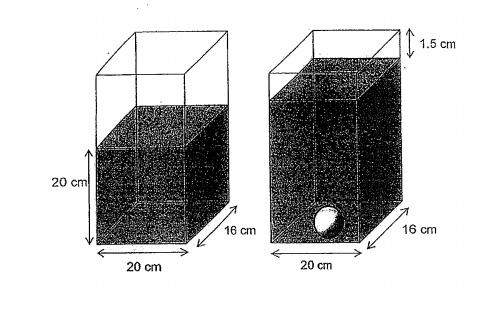# Question 1 of 50

A tank was $\frac{2}{3}$ filled with water to a height of 20cm.
(a) What is the capacity of the tank?
(b) When a glass ball was dropped into the tank, the height of the water level was 1.5cm from the brim of the tank. Find the volume of the glass ball.A
(a) 8000
(b) 264cm$^3$
B
(a) 8800
(b) 268cm$^3$
C
(a) 9200
(b) 270cm$^3$
D
(a) 9600
(b) 272cm$^3$
E
None of the above